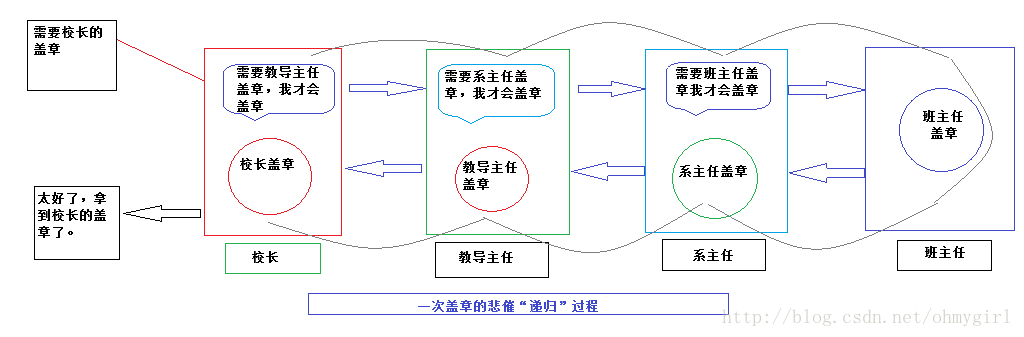# PHP中递归的实现实例详解

2018-09-07 11:49

递归的定义

递归（wiki/Recursive）是一种函数调用自身（直接或间接）的一种机制，这种强大的思想可以把某些复杂的概念变得极为简单。在计算机科学之外，尤其是在数学中，递归的概念屡见不鲜。例如：最常用于递归讲解的斐波那契数列便是一个极为典型的例子，而其他的例如阶层（n!）也可以转化为递归的定义（n! = n*(n-1)!）.即使是在现实生活中，递归的思想也是随处可见：例如，由于学业问题你需要校长盖章，然而校长却说“只有教导主任盖章了我才会盖章”，当你找到教导主任，教导主任又说：“只有系主任盖章了我才会盖章”...直到你最终找到班主任，在得到班主任豪爽的盖章之后，你要依次返回到系主任、教导主任、最后得到校长的盖章，过程如下：递归函数是一种调用自己的函数。写递归函数时要小心，因为可能会无穷递归下去。必须确保有充分的方法来终止递归。

一：使用 参数引用 完成递归函数。操作的是同一块内存地址。

<?php \$i=1; function test(&\$i) { echo \$i; \$i++; if (\$i < 10) { test(\$i); } } test(\$i);// 输出123456789 test ( \$i );// 输出10 ?>

二：使用 全局变量 完成递归函数。

在函数域内部用 global 语句导入的一个真正的全局变量实际上是建立了一个到全局变量的引用。例子中，test()函数内部的 \$i 实际上只是程序第一行中（\$i = 1;）的变量 \$i 的一个应用；

<?php \$i = 1 ; function test () { global \$i ; echo \$i ; \$i++; if (\$i <10 ) { test(); } } test();// 输出123456789 test ();// 输出10 ?>

三：使用 静态变量 完成递归函数。

static的作用：仅在第一次调用函数的时候对变量进行初始化，并且保留变量值。

<?php function test () { static \$i = 1 ; echo \$i ; \$i ++; if ( \$i < 10 ) { test (); } \$i --;// 在每一层递归结束时自减，这一句可以帮助理解递归函数的执行过程 } test();// 输出123456789 test();// 输出123456789 ?>

例1. 使用全局变量的情况 递归遍历文件夹下的所有文件

function getFiles(\$dir) { global \$arr; if(is_dir(\$dir)){ \$hadle = @opendir(\$dir); while(\$file=readdir(\$hadle) ) { if(!in_array(\$file,array(., ..)) ) { \$dirr = \$dir./.\$file; if(is_dir(\$dirr)) { getFiles(\$dirr); }else{ array_push(\$arr, \$dirr); } } } } } \$arr = array(); getFiles(E:/logs); print_r(\$arr);

例2：使用静态变量的情况递归遍历文件夹下的所有文件

function getFiles (\$dir) { static \$arr = array(); if(is_dir(\$dir)){ \$hadle = opendir(\$dir); while(\$file=readdir(\$hadle)) { if(!in_array(\$file,array(.,..)) ) { \$dirr = \$dir./.\$file; if(is_dir(\$dirr)) { getFiles (\$dirr); }else{ array_push(\$arr,\$dirr); } } } } return \$arr; } \$rows= array(); \$rows = getFiles (E:/logs); print_r(\$rows);

总结

以上所述是小编给大家介绍的PHP中递归的实现实例详解，希望对大家有所帮助，如果大家有任何疑问请给我留言，小编会及时回复大家的。在此也非常感谢大家对脚本之家网站的支持！